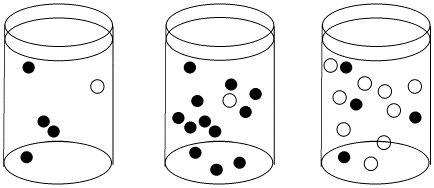# Kinetics of Assoc. Mechanism

We can measure the rate of an associative reaction and make changes in the reaction conditions to see how the rate is affected. For example, we could easily change the concentrations of the two reactants. All we have to do is change the amount of reactant we dissolve in the solution.

If we did that, we would find a linear relationship between each concentration and rate. If we double the concentration of new ligand, the rate of reaction doubles. If we triple it, the rate triples. Also, if we double the amount of metal complex, the rate doubles and so on. We can write the following expression, called the rate law, to describe this relationship:

$\text {Rate Law: Rate} = {-d[MLn]\over dt } = k[MLn][L']$

This type of reaction is sometimes called a second order reaction. That term just refers to the mathematical form of the rate law, which depends on concentration times concentration, or concentration squared. The "order" of the reaction is the number of concentrations multiplied together in the rate law.

Why does the associative mechanism depend on concentrations in this specific way?

This is a case of two molecules coming together. If both compounds are dissolved in solution, they must “swim around” or travel through the solution until they bump into each other and react. The more concentrated the solution is, or the more crowded it is with molecules, the more likely are the reactants to bump into each other. If we double the amount of new ligand in solution, an encounter between ligand and complex becomes twice as likely. If we double the amount of metal complex in solution, an encounter also becomes twice as likely.Figure LS3.1. The effect of concentration on collision probability. In the first beaker, there is a chance that a black molecule and white molecule will meet and react together. The chance of a meeting is much higher in both the second beaker, where there are lots more black molecules, and in the third beaker, where there are many more white molecules.

### Problem LS3.1.

Given the associative rate law above, what would happen to the reaction rate for an associative substitution in the following cases?

1. the concentration of ligand is doubled, and the concentration of metal complex is doubled
2. the concentration of ligand is tripled, and the concentration of metal complex is doubled
3. the concentration of ligand is tripled, and the concentration of metal complex is tripled
4. the concentration of ligand is halved, and the concentration of metal complex is doubled

### Problem LS3.2.

Plot graphs of initial rate vs. concentration to show what you would see in associative substitution.

1. The concentration of new ligand, [X], is held constant at 0.1 mol/L and the concentration of metal complex is changed from 0.5 mol/L to 1 mol/L and then to 1.5 mol/L.
2. The concentration of metal complex, [MLn], is held constant at 0.1 mol/L and the concentration of ligand is changed from 0.5 mol/L to 1 mol/L and then to 1.5 mol/L.

### Problem LS3.3.

In the previous problem, the experiment was run in a particular way for particular reasons.

1. Why was one concentration held constant while the other one was changed? Why not change both?
2. Why does the graph report "initial rate" -- just the rate at the very beginning of the reaction?

### Problem LS3.5.

What information can be gained from the slopes of lines in Problem LS3.4.?